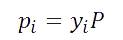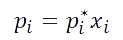### Flow Rate

#### Standard to Actual: Gas Flow

${\frac{{\mathrm{ft}}^{3}}{\mathrm{hr}}}_{\left[S\right]}={\frac{{\mathrm{ft}}^{3}}{\mathrm{hr}}}_{\left[A\right]}×\frac{520}{T+460}×\frac{P+14.7}{14.7}$

$T=\text{Temperature}\phantom{\rule{5px}{0ex}}\left[°\mathrm{F\right]}\phantom{\rule{0ex}{0ex}}P=\text{Pressure}\phantom{\rule{5px}{0ex}}\mathrm{\left[psig\right]}$

### Pressure

$P=\frac{\mathrm{SG}×H}{2.31}$

### Viscosity

$\gamma =\frac{\Phi }{\mathrm{SG}}$

$\gamma =\text{Kinematic Viscosity}\phantom{\rule{5px}{0ex}}\mathrm{\left[cSt\right]}\phantom{\rule{0ex}{0ex}}\Phi =\text{Dynamic Viscosity}\phantom{\rule{5px}{0ex}}\mathrm{\left[cP\right]}\phantom{\rule{0ex}{0ex}}\mathrm{SG}=\text{Specific Gravity}$

### API

$\mathrm{API}=\left(\frac{141.5}{\mathrm{SG}}\right)-131.5$### Partial Pressure

The Product of the total pressure P, of the gas, times the mole fraction of that species y(i) in the gas.Raoult's Law

The partial vapor pressure of each component of an ideal mixture of liquids is equal to the vapor pressure of the pure component multiplied by its mole fraction in the mixture.

For a single component in an ideal solution:### ​$K=\frac{{C}_{P}}{{C}_{P}-\frac{R}{\mathrm{MW}}}$### General Reference

• Neutrium
Neutrium is a knowledge base of engineering topics, centered mainly around chemical engineering design challenges faced by engineers in their daily work. We created Neutrium to bridge the gap between theory and practice. Feel free to ask a question, leave feedback or take a look at one of our in-depth articles.
• PetroWiki
PetroWiki was created from the seven volume Petroleum Engineering Handbook (PEH) published by the Society of Petroleum Engineers (SPE).
• Thermopedia
THERMOPEDIA is the gateway to resources on heat and mass transfer, fluid flow and thermodynamics.
• Statistical Distribution